Courses

# Past Year Questions: Heat Transfer In Flow Over Plates And Pipes

## 15 Questions MCQ Test Engineering Mechanics | Past Year Questions: Heat Transfer In Flow Over Plates And Pipes

Description
This mock test of Past Year Questions: Heat Transfer In Flow Over Plates And Pipes for Mechanical Engineering helps you for every Mechanical Engineering entrance exam. This contains 15 Multiple Choice Questions for Mechanical Engineering Past Year Questions: Heat Transfer In Flow Over Plates And Pipes (mcq) to study with solutions a complete question bank. The solved questions answers in this Past Year Questions: Heat Transfer In Flow Over Plates And Pipes quiz give you a good mix of easy questions and tough questions. Mechanical Engineering students definitely take this Past Year Questions: Heat Transfer In Flow Over Plates And Pipes exercise for a better result in the exam. You can find other Past Year Questions: Heat Transfer In Flow Over Plates And Pipes extra questions, long questions & short questions for Mechanical Engineering on EduRev as well by searching above.
QUESTION: 1

### For the fluid flowing over a flat plate with Prandtl number greater than unity, the thermal boundary layer for laminar forced convection. 

Solution: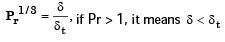QUESTION: 2

### A fluid flowing over a flat plate has the following properties: dynamic viscosity = 25 × 10–6 kg/ ms, specific heat = 2.0 kJ/kgK, thermal conductivity 0.05 W/mK. The hydrodynamic boundary layer thickness is measured to be 0.5 mm. The thickness of the thermal boundary layer would be 

Solution: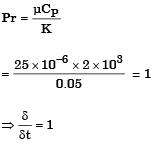Now, δ = 0.5 mm
∴ δ = δt = 0.5mm

QUESTION: 3

### Water (Prandtl number = 6) flows over a flat plate which is heated over the entire length. Which one of the following relationship between the hydrodynamic boundary layer thickness (δ) and thermal boundary layer thickness (δt) is true 

Solution: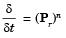Here, Pr = 6 > 1
or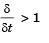∴ δ > δt

QUESTION: 4

Consider a laminar boundary layer over a heated flat plate. The free stream velocity is U. At some distance x from the leading edge the velocity boundary layer thickness is δv and the thermal boundary layer thickness is δT. If the Prandtl number is greater than 1, then



Solution:

Prandtl number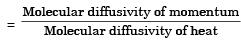From question, since prandtl number > 1
∴ Velocity boundry thickness (δv) > thermal boundary thickness (δt)

QUESTION: 5

An un-insulated air conditioning duct of rectangular cross section 1 m × 0.5 m, carrying air at 20°C with a velocity of 10 m/s, is exposed to an ambient of 30°C. Neglect the effect of duct construction material. For air in the range of 20 – 30°C, data is as follows: thermal conductivity = 0.025 W/mK; viscosity = 18 μPa.s; Prandtl number = 0.73; density =1.2 kg/m3. For laminar flow Nusselt number is 3.4 for constant wall temperature conditions and , for turbulent flow, Nu = 0.023 Re0.8Pr0.33.The Reynolds number for the flow is



Solution: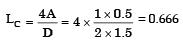Reynolds number,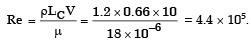QUESTION: 6

An un-insulated air conditioning duct of rectangular cross section 1 m × 0.5 m, carrying air at 20°C with a velocity of 10 m/s, is exposed to an ambient of 30°C. Neglect the effect of duct construction material. For air in the range of 20 – 30°C, data is as follows: thermal conductivity = 0.025 W/mK; viscosity = 18 μPa.s; Prandtl number = 0.73; density =1.2 kg/m3. For laminar flow Nusselt number is 3.4 for constant wall temperature conditions and , for turbulent flow, Nu = 0.023 Re0.8Pr0.33.

The heat transfer per meter length of the duct, in watts, is



Solution:

As Re > 4000, the flow is turbulent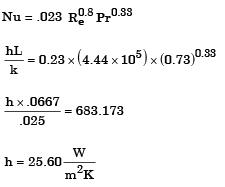Surface area of duct
A = 2 × al + 2 × bl
where, l = length of duct
∴ A = 3l
Heat transfer rate, Q = h A(T0 – T)
Q = 25.60 × 3l × (30 – 20)
Q/I = 769W

QUESTION: 7

The temperature distribution with in the thermal boundary layer over a heated isothermal flat plate is given by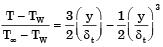where TW and T are the temperatures of plate and free stream respectively, and y is the normal distance measured from the plate. The local Nusselt number based on the thermal boundary layer thickness δt is given by



Solution:

Only (b) satisfies the conditions

QUESTION: 8

For flow of fluid over a heated plate , the following fluid properties are known: viscosity = 0.001 Pa.s; specific heat at constant pressure = 1 kJ/kgK; thermal conductivity = 1 W/mK.
The hydrodynamic boundary layer thickness at a specified location on the plate is 1 mm. The thermal boundary layer thickness at the same location is



Solution:

Given: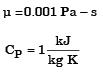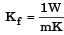(Fluid thermal conductivity)
Hydrodynamic boundary layer thickness, δ = 1 mm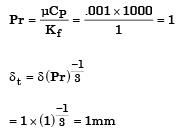QUESTION: 9

A pipe of 25 mm outer diameter carries steam. The heat transfer coefficient between the cylinder and surroundings is 5 W/m2K. It is proposed to reduce the heat loss from the pipe by adding insulation having a thermal conductivity of 0.05 W/mK. Which one of the following statements is TRUE?



Solution:

Critical radius of insulation = (k/h)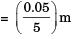= 10mm
(router) > rcritical
Thus, adding insulation shall decrease H.T. Rate.

QUESTION: 10

The ratios of the laminar hydrodynamic boundary layer thickness to thermal boundary layer thickness of flows of two fluids P and Q on a flat plate are 1/2 and 2 respectively. The Reynolds number based on the plate length for both the flows is 104. The Prandtl and Nusselt numbers for P are 1/8 and 35 respectively. The Prandlt and Nusselt numbers for Q are respectively



Solution: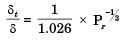For fluid Q :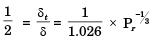⇒ Pr = 8
For fluid P :  Laminar flow over flat plate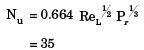Similarly for fluid Q: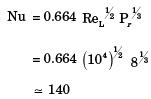QUESTION: 11

Consider a two-dimensional laminar flow over a long cylinder as shown in the figure below.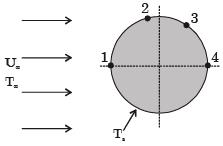The free stream velocity is U and the freestream temperature T is lower than the cylinder surface temperature T. The local heat transfer coefficient is minimum at point



Solution:

For laminar flow, the heat transfer coefficient is minimum where the boundary layer thickness is maximum and vice versa. For turbulentregion boundary layer thickness is maximum at 3 but for laminar boundary layer thickness is maximum at 2 so minimum heat transfer coefficient.

QUESTION: 12

The non-dimensional fluid temperature profile near the surface of a convectively cooled flat plate is given by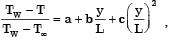where y is measured perpendicular to the plate, L is the plate length, and a, b and c are arbitrary constants. TW and T are wall and ambient temperatures, respectively. If the thermal conductivity of the fluid is k and the wall heat flux is q', the Nusselt number Nu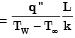is equal to



Solution: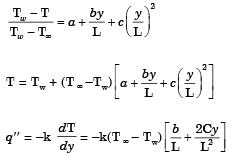at y = 0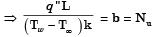∴ Nu = b.

QUESTION: 13

For flow of viscous fluid over a flat plate, if the fluid temperature is the same as the plate temperature, the thermal boundary layer is



Solution:
QUESTION: 14

The Blausius equation related to boundary layer theory is a



Solution:

Blausius equation :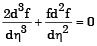This order non-linear D.E.

QUESTION: 15

For flow through a pipe of radius R, the velocity and temperature distribution are as follows: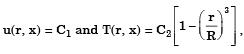where C1 and C2 are constants. The bulk temperature is given by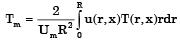with Um being the mean velocity of flow. The value of Tm is



Solution: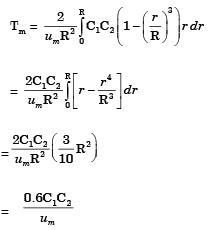Since u(r, x) is constant, um = C1
∴ tm = 0.6C2